# Same-Side Interior Angles: Theorem, Proof, and Examples

• Author:
• Updated date:

Ray is a licensed engineer in the Philippines. He loves to write about mathematics and civil engineering.

The same-side interior angles are two angles that are on the same side of the transversal line and in between two intersected parallel lines. A transversal line is a straight line that intersects one or more lines.

The Same-Side Interior Angles Theorem states that if a transversal cuts two parallel lines, then the interior angles on the same side of the transversal are supplementary. Supplementary angles are ones that have a sum of 180°.

Same-Side Interior Angles Theorem Proof

Let L1 and L2 be parallel lines cut by a transversal T such that ∠2 and ∠3 in the figure below are interior angles on the same side of T. Let us show that ∠2 and ∠3 are supplementary.

Since ∠1 and ∠2 form a linear pair, then they are supplementary. That is, ∠1 + ∠2 = 180°. By the Alternate Interior Angle Theorem, ∠1 = ∠3. Thus, ∠3 + ∠2 = 180°. Therefore, ∠2 and ∠3 are supplementary.

## The Converse of Same-Side Interior Angles Theorem

If a transversal cuts two lines and a pair of interior angles on the same side of the transversal is supplementary, then the lines are parallel.

The Converse of Same-Side Interior Angles Theorem Proof

Let L1 and L2 be two lines cut by transversal T such that ∠2 and ∠4 are supplementary, as shown in the figure. Let us prove that L1 and L2 are parallel.

Since ∠2 and ∠4 are supplementary, then ∠2 + ∠4 = 180°. By the definition of a linear pair, ∠1 and ∠4 form a linear pair. Thus, ∠1 + ∠4 = 180°. Using the transitive property, we have ∠2 + ∠4 = ∠1 + ∠4. By the addition property, ∠2 = ∠1

Hence, L1 is parallel to L2.

## Example 1: Finding the Angle Measures Using Same-Side Interior Angles Theorem

In the accompanying figure, segment AB and segment CD, ∠D = 104°, and ray AK bisect ∠DAB. Find the measure of ∠DAB, ∠DAK, and ∠KAB.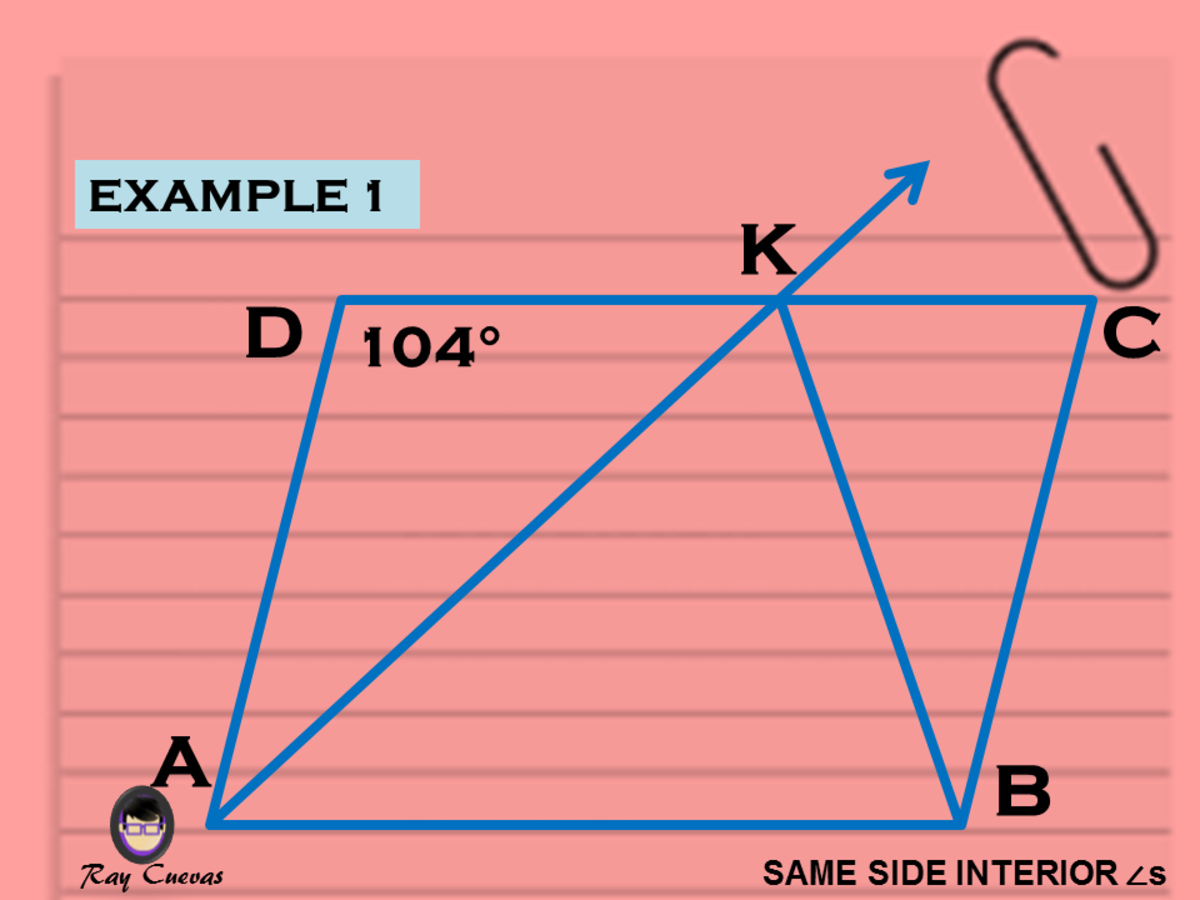Example 1: Finding the Angle Measures Using Same-Side Interior Angles TheoremJohn Ray Cuevas
Scroll to Continue

Solution

Since side AB and CD are parallel, then the interior angles, ∠D and ∠DAB, are supplementary. Thus, ∠DAB = 180° - 104° = 76°. Also, since ray AK bisects ∠DAB, then ∠DAK ≡ ∠KAB.

Therefore, ∠DAK = ∠KAB = (½)(76) = 38.

## Example 2: Determining if Two Lines Cut by Transversal Are Parallel

Identify if lines A and B are parallel given the same-side interior angles, as shown in the figure below.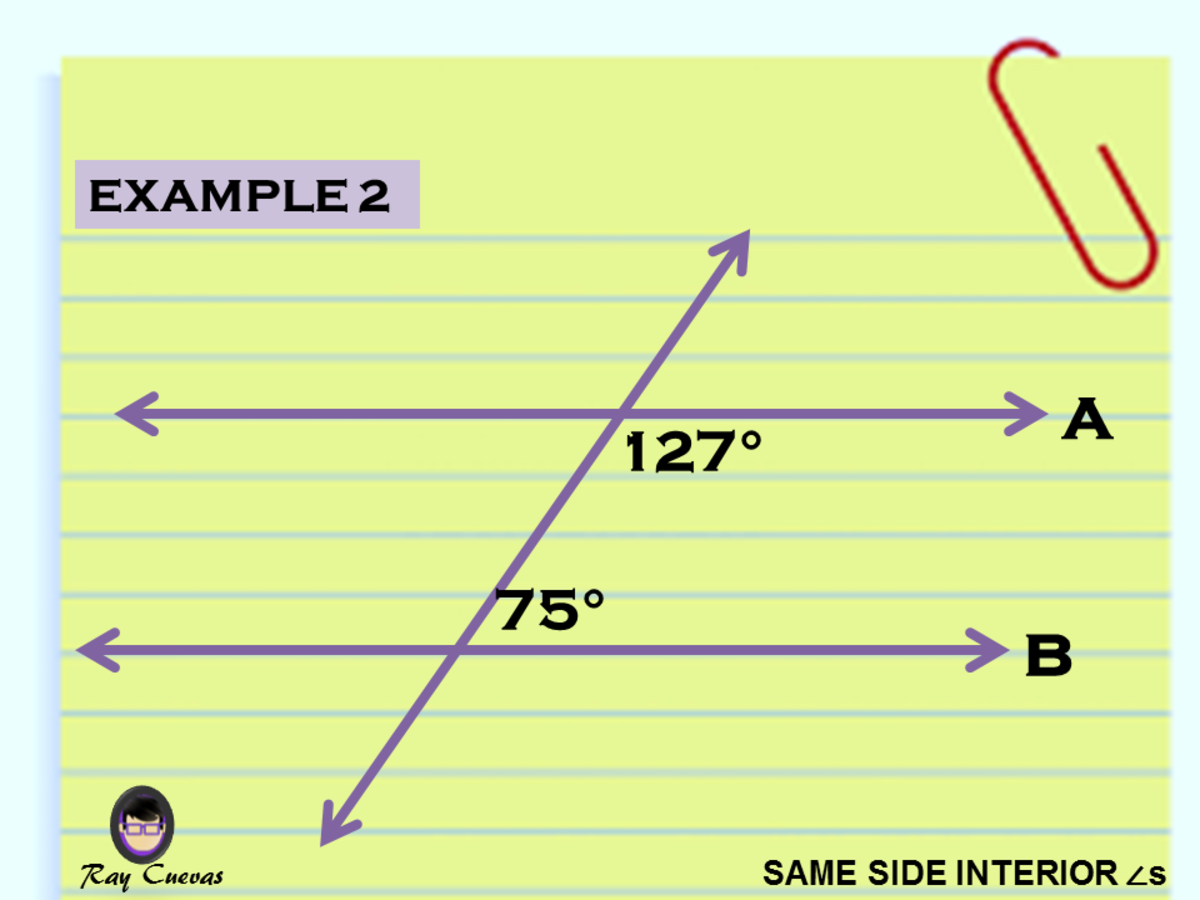Example 2: Determining if Two Lines Cut by Transversal Are ParallelJohn Ray Cuevas

Solution

Apply the Same-Side Interior Angles Theorem in finding out if line A is parallel to line B. The theorem states that the same-side interior angles must be supplementary given the lines intersected by the transversal line are parallel. If the two angles add up to 180°, then line A is parallel to line B.

127° + 75° = 202°

Since the sum of the two interior angles is 202°, therefore the lines are not parallel.

## Example 3: Finding the Value of X of Two Same-Side Interior Angles

Find the value of x that will make L1 and L2 parallel.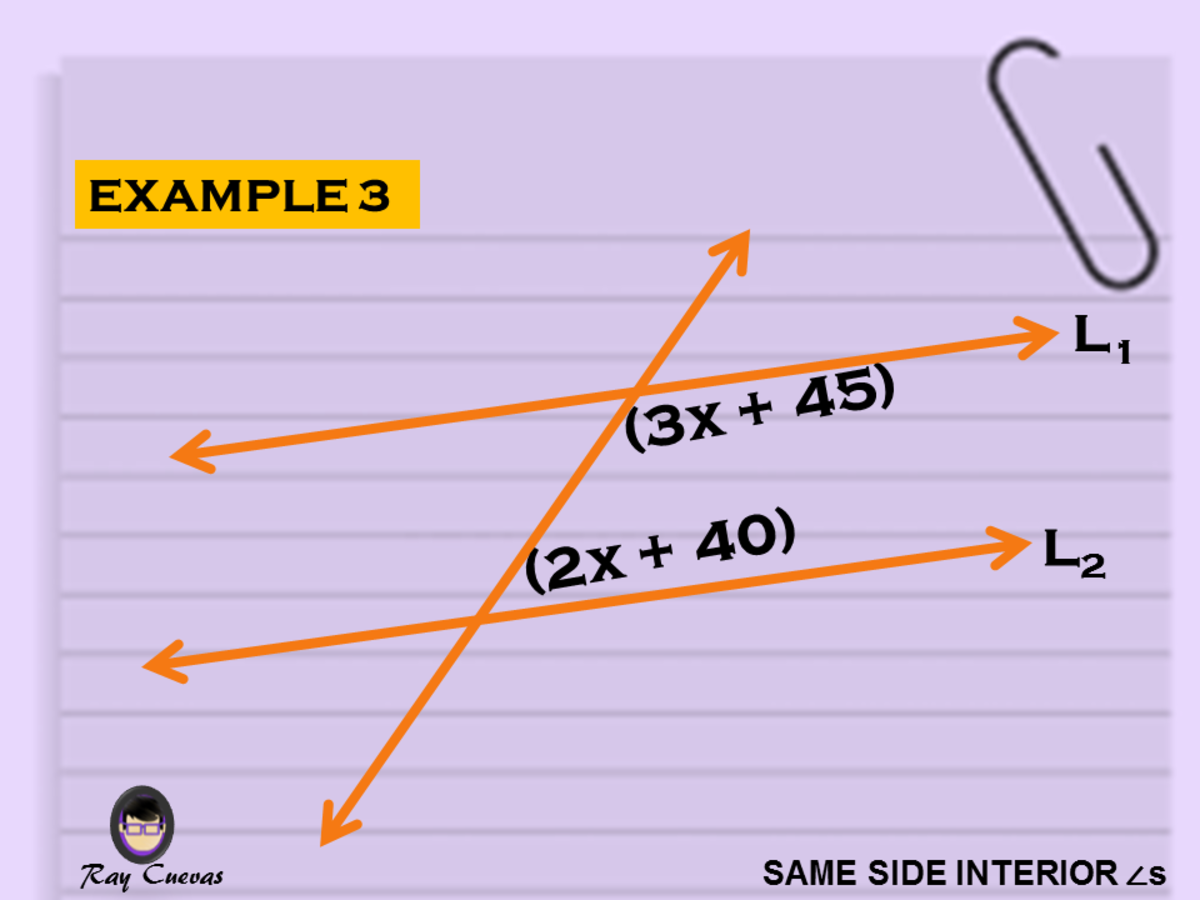Example 3: Finding the Value of X of Two Same-Side Interior AnglesJohn Ray Cuevas

Solution

The given equations are the same-side interior angles. Since the lines are considered parallel, the angles’ sum must be 180°. Make an expression that adds the two equations to 180°.

(3x + 45) + (2x + 40) = 180

5x + 85 = 180

5x = 180 – 85

5x = 95

x = 19

The final value of x that will satisfy the equation is 19.

## Example 4: Finding the Value of X Given Equations of the Same-Side Interior Angles

Find the value of x given m∠4 = (3x + 6)° and m∠6 = (5x + 12)°.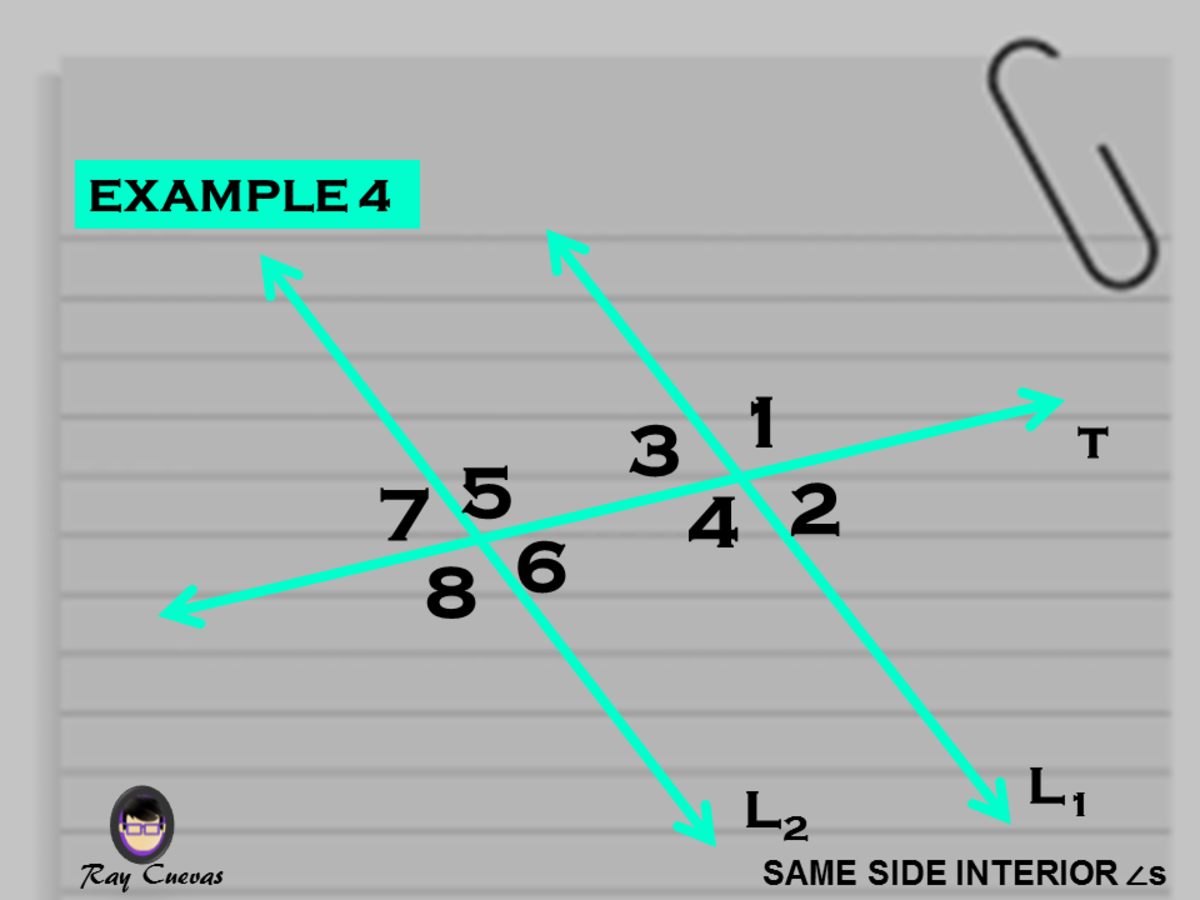Example 4: Finding the Value of X Given Equations of the Same-Side Interior AnglesJohn Ray Cuevas

Solution

The given equations are the same-side interior angles. Since the lines are considered parallel, the angles’ sum must be 180°. Make an expression that adds the expressions of m∠4 and m∠6 to 180°.

m∠4 + m∠4 = 180

3x + 6 + 5x + 12 = 180

8x + 20 = 180

8x = 180 - 20

8x = 160

x = 20

The final value of x that will satisfy the equation is 20.

## Example 5: Finding the Value of Variable Y Using Same-Side Interior Angles Theorem

Solve for the value of y given its angle measure is the same-side interior angle with the 105° angle.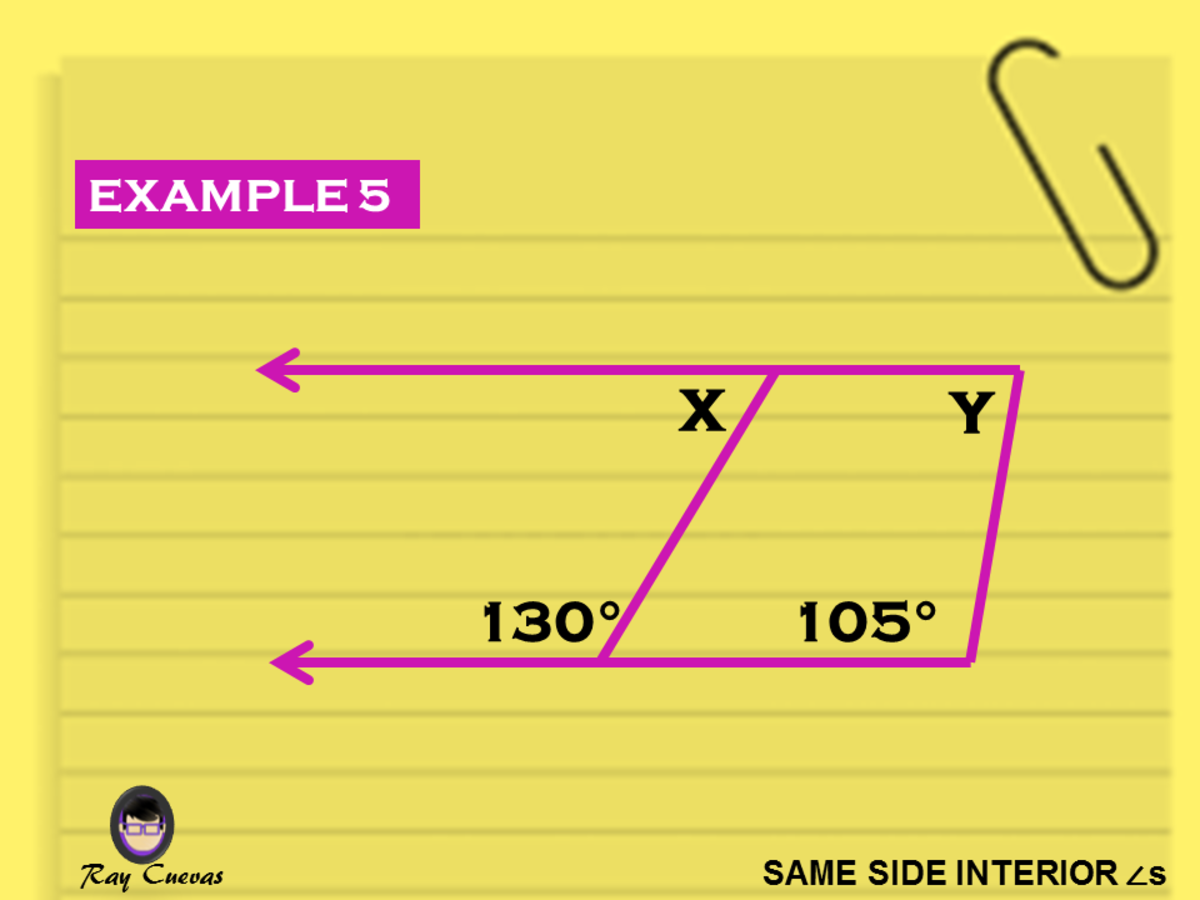Example 5: Finding the Value of Variable Y Using Same-Side Interior Angles TheoremJohn Ray Cuevas

Solution

See to it that y and the obtuse angle 105° are same-side interior angles. It simply means that these two must equate to 180° to satisfy the Same-Side Interior Angles Theorem.

y + 105 = 180

y = 180 – 105

y = 75

The final value of x that will satisfy the theorem is 75.

## Example 6: Finding the Angle Measure of All Same-Side Interior Angles

The lines L1 and L2 in the diagram shown below are parallel. Find the angle measures of m∠3, m∠4, and m∠5.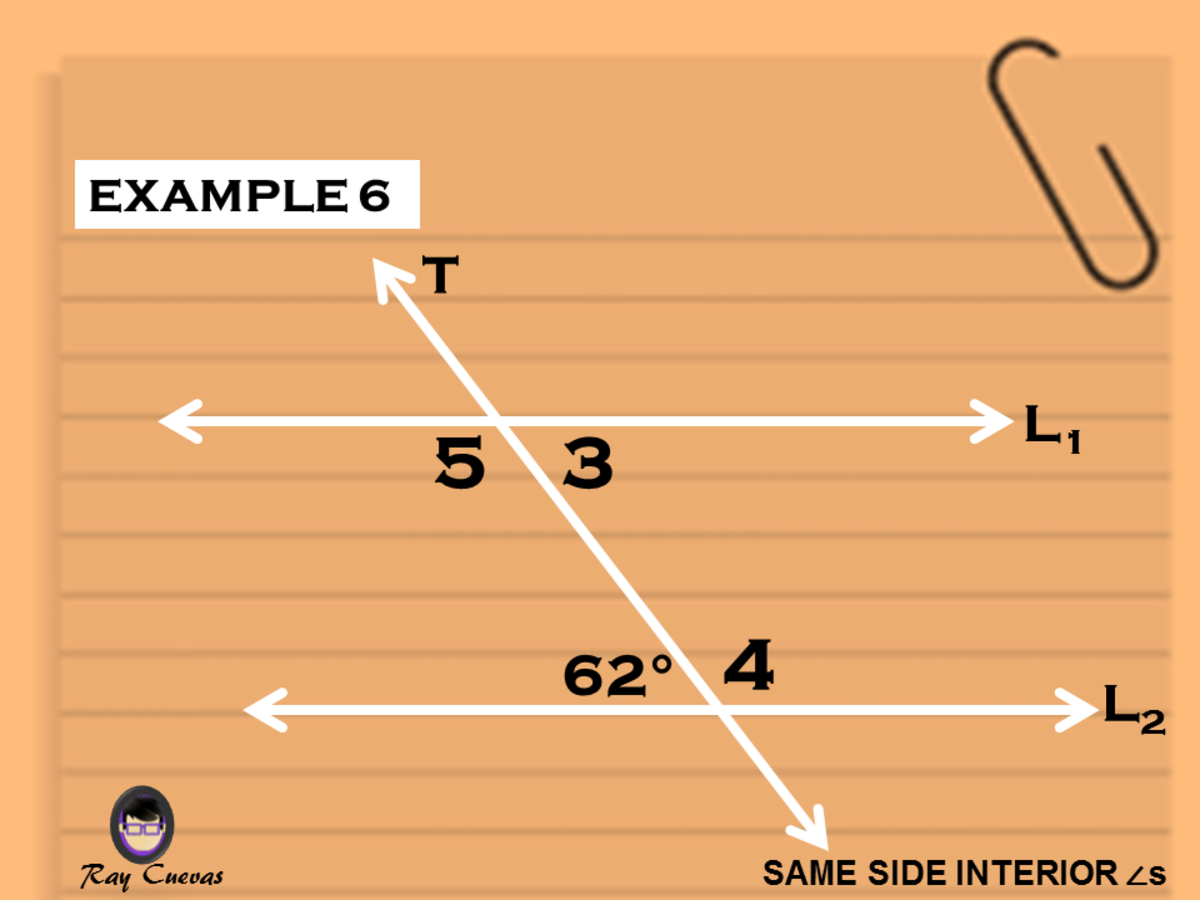Example 6: Finding the Angle Measure of All Same-Side Interior AnglesJohn Ray Cuevas

Solution

The lines L1 and L2 are parallel, and according to the Same-Side Interior Angles Theorem, angles on the same side must be supplementary. Note that m∠5 is supplementary to the given angle measure 62°, and

m∠5 + 62 = 180

m∠5 = 180 – 62

m∠5 = 118

Since m∠5 and m∠3 are supplementary. Make an expression adding the obtained angle measure of m∠5 with m∠3 to 180.

m∠5 + m∠3 = 180

118 + m∠3 = 180

m∠3 = 180 – 118

m∠3 = 62

The same concept goes for the angle measure m∠4 and the given angle 62°. Equate the sum of the two to 180.

62 + m∠4 = 180

m∠4 = 180 – 62

m∠4 = 118

It also shows that m∠5 and m∠4 are angles with the same angle measure.

m∠5 = 118°, m∠3 = 62°, m∠4 = 118°

## Example 7: Proving Two Lines Are Not Parallel

The lines L1 and L2, as shown in the picture below, are not parallel. Describe the angle measure of z?

Solution

Given that L1 and L2 are not parallel, it is not allowed to assume that angles z and 58° are supplementary. The value of z cannot be 180° - 58° = 122°, but it could be any other measure of higher or lower measure. Also, it is evident with the diagram shown that L1 and L2 are not parallel. From there, it is easy to make a smart guess.

The angle measure of z = 122°, which implies that L1 and L2 are not parallel.

## Example 8: Solving for the Angle Measures of Same-Side Interior Angles

Find the angle measures of ∠b, ∠c, ∠f, and ∠g using the Same-Side Interior Angle Theorem, given that the lines L1, L2, and L3 are parallel.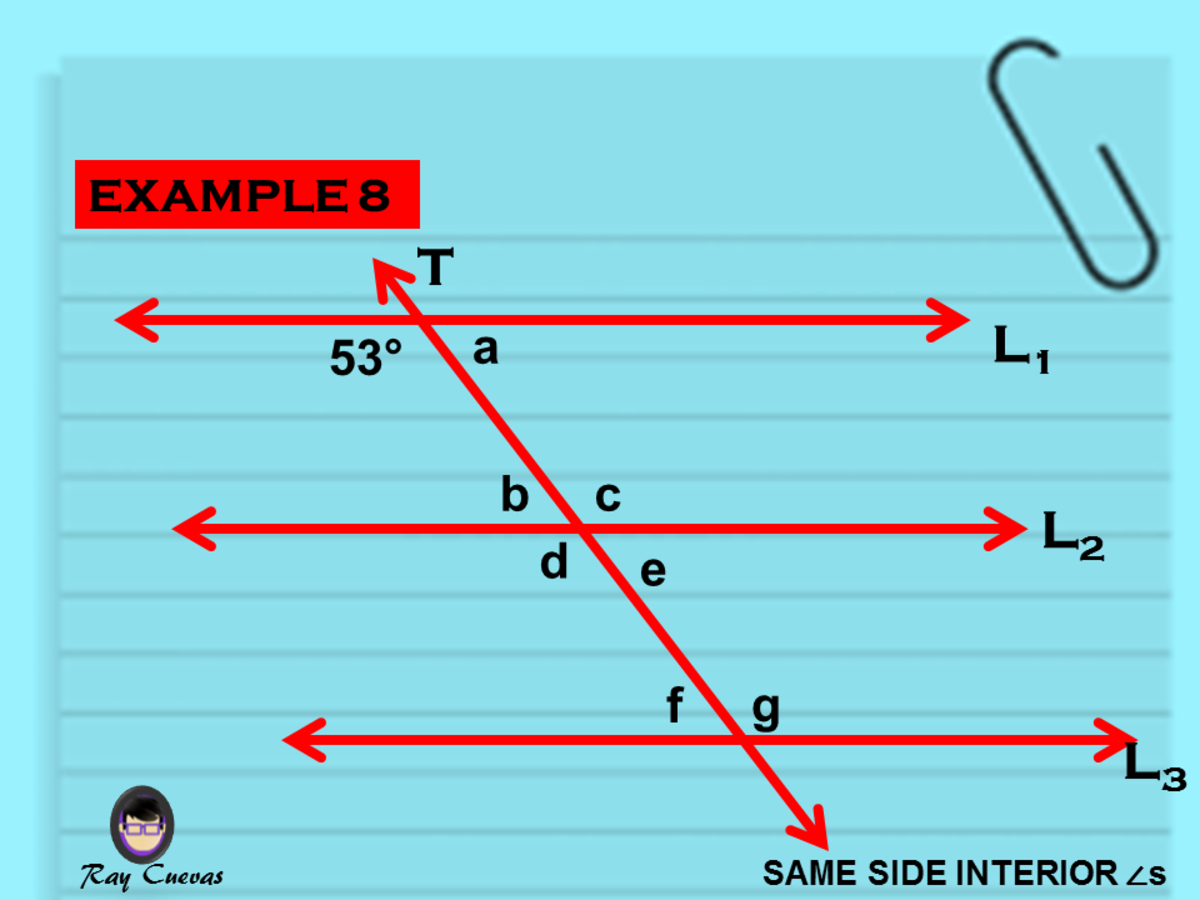Example 8: Solving for the Angle Measures of Same-Side Interior AnglesJohn Ray Cuevas

Solution

Given that L1 and L2 are parallel, m∠b and 53° are supplementary. Create an algebraic equation showing that the sum of m∠b and 53° is 180°.

m∠b + 53 = 180

m∠b = 180 – 53

m∠b = 127

Since the transversal line cuts L2, therefore m∠b and m ∠c are supplementary. Make an algebraic expression showing that the sum of ∠b and ∠c is 180°. Substitute the value of m∠b obtained earlier.

m∠b + m∠c = 180

127 + m∠c = 180

m∠c = 180 – 127

m∠c = 53

Since the lines L1, L2, and L3 are parallel, and a straight transversal line cuts them, all the same-side interior angles between the lines L1 and L2 are the same with the same-side interior of L2 and L3.

m∠f = m∠b

m∠f = 127

m∠g = m∠c

m∠g = 53

m∠b = 127°, m∠c = 53°, m∠f = 127°, m∠g = 53°

## Example 9: Identifying the Same-Side Interior Angles in a Diagram

Give the complex figure below; identify three same-side interior angles.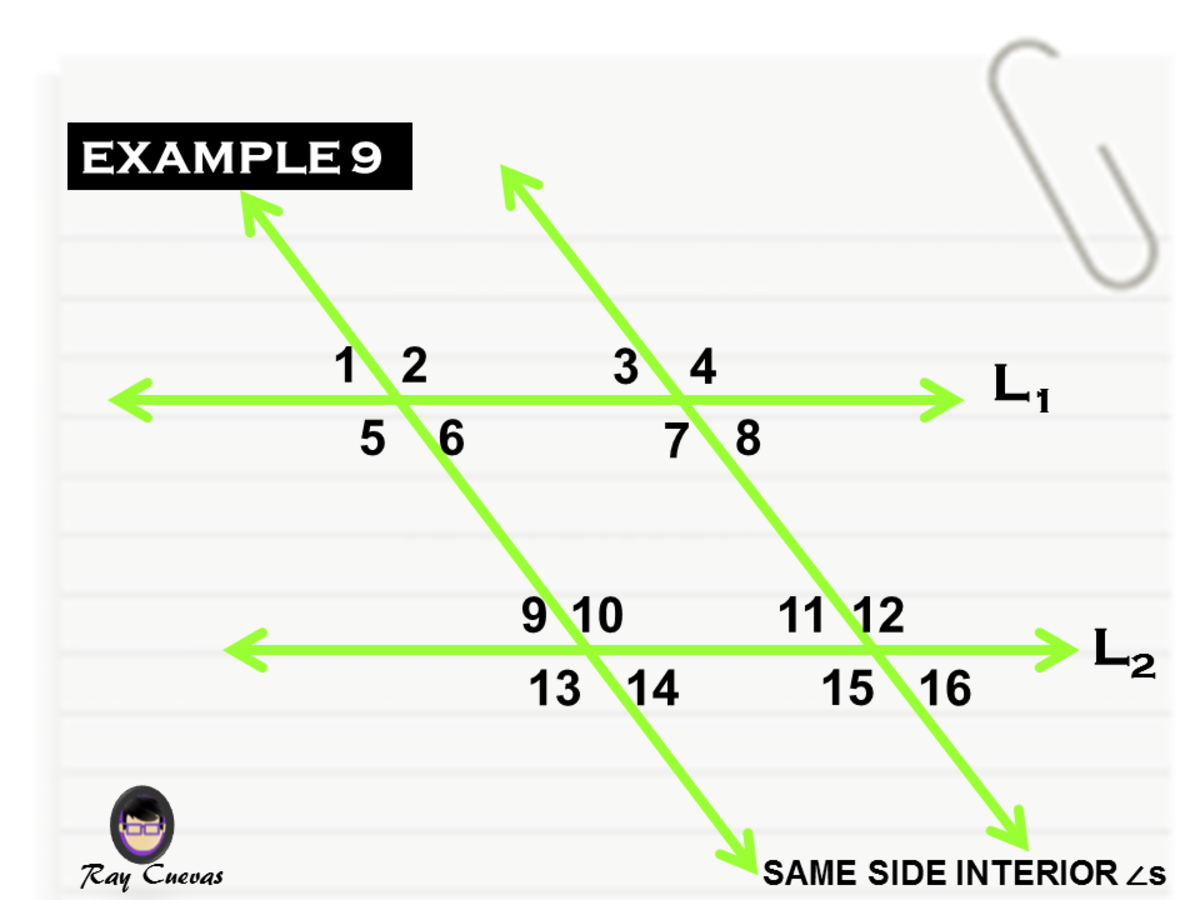Example 9: Identifying the Same-Side Interior Angles in a DiagramJohn Ray Cuevas

Solution

There are a lot of same-side interior angles present in the figure. Through keen observation, it is safe to infer that three out of many same-side interior angles are ∠6 and ∠10, ∠7 and ∠11, and ∠5 and ∠9.

## Example 10: Determining Which Lines Are Parallel Given a Condition

Given ∠AFD and ∠BDF are supplementary, determine which lines in the figure are parallel.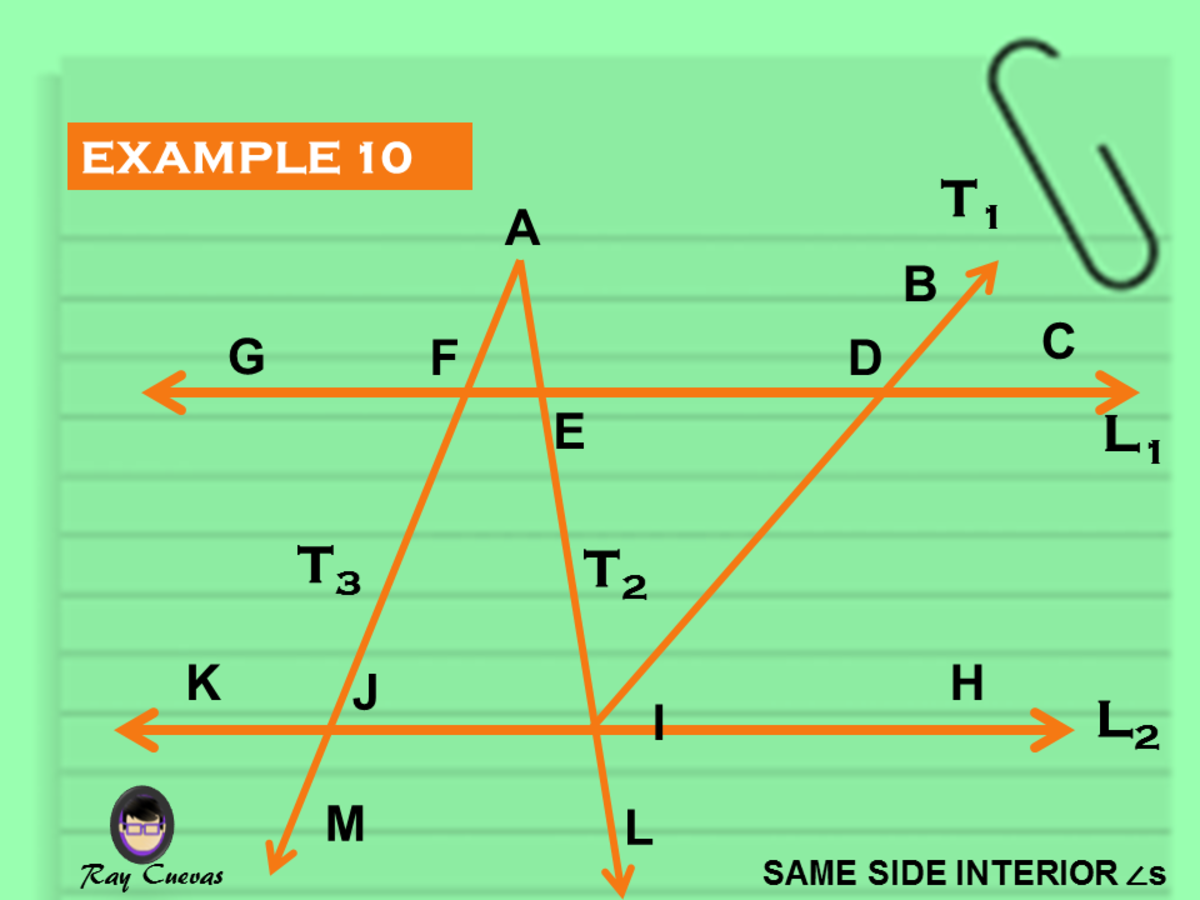Example 10: Determining Which Lines Are Parallel Given a ConditionJohn Ray Cuevas

Solution

By keen observation, given the condition that ∠AFD and ∠BDF are supplementary, the parallel lines are line AFJM and line BDI.

## Explore Other Math Articles

This content is accurate and true to the best of the author’s knowledge and is not meant to substitute for formal and individualized advice from a qualified professional.